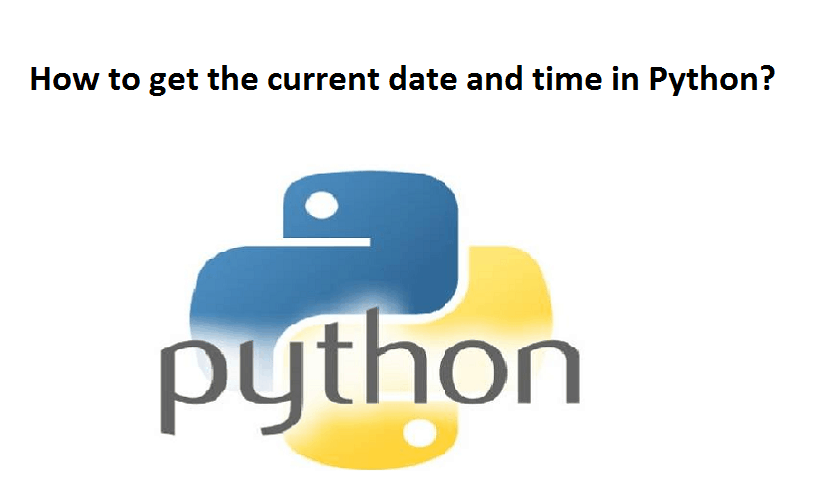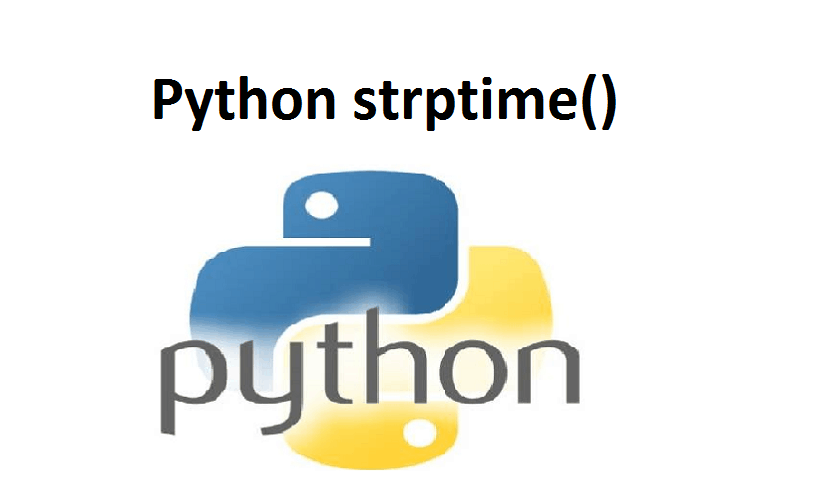# How to get the current date and time in Python?In this article, you will learn to get today’s date and current date and time in Python. We will also formate the date and time in various formats using the strftime() method.

There are various ways you can take to get the current date. We will use the date class of the datetime module to accomplish this task.

## Example 1: Python get today’s date

```from datetime import date

today = date.today()
print("Today's date:", today)```

Here, we imported the date class from the datetime module. Then, we used the date.today() method to get the current local date.

By the way, date.today() returns a date object, which is assigned to the today variable in the above program. Now, you can use the strftime() method to create a string representing a date in different formats.

## Example 2: Current date in different formats

```from datetime import date

today = date.today()

# dd/mm/YY
d1 = today.strftime("%d/%m/%Y")
print("d1 =", d1)

# Textual month, day and year
d2 = today.strftime("%B %d, %Y")
print("d2 =", d2)

# mm/dd/y
d3 = today.strftime("%m/%d/%y")
print("d3 =", d3)

# Month abbreviation, day and year
d4 = today.strftime("%b-%d-%Y")
print("d4 =", d4)```

When you run the program, the output will be something like:

```d1 = 16/09/2019
d2 = September 16, 2019
d3 = 09/16/19
d4 = Sep-16-2019
```

If you need to get the current date and time, you can use the datetime class of the datetime module.

## Example 3: Get the current date and time

```from datetime import datetime

# datetime object containing current date and time
now = datetime.now()

print("now =", now)

# dd/mm/YY H:M:S
dt_string = now.strftime("%d/%m/%Y %H:%M:%S")
print("date and time =", dt_string)	```

Here, we have used datetime.now() to get the current date and time. Then, we used strftime() to create a string representing date and time in another format.

Please feel free to give your comment if you face any difficulty here.

For more Articles click on the below link.### Python strptime()### Python Get Current time